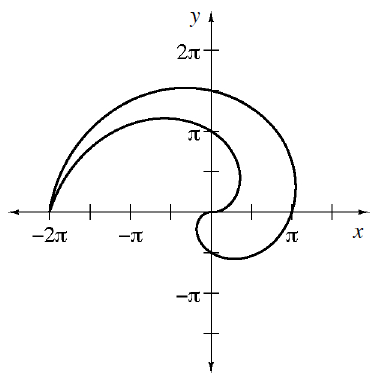### Home > APCALC > Chapter 11 > Lesson 11.3.1 > Problem11-81

11-81.

Calculate the area of the region bounded by the curves $r = \left(2θ\right)$ and $r = –θ$. A graph of this region is shown to the right.. .Determine the bounds for each curve.

$A=\int_0^{2\pi}\frac{1}{2}(-\theta)^2d\theta-\int_0^{\pi}\frac{1}{2}(2\theta)^2d\theta$

Use the eTool below to help solve the problem.
Click on the link to the right to view the full version of the eTool. Calc 8-81 HW eTool